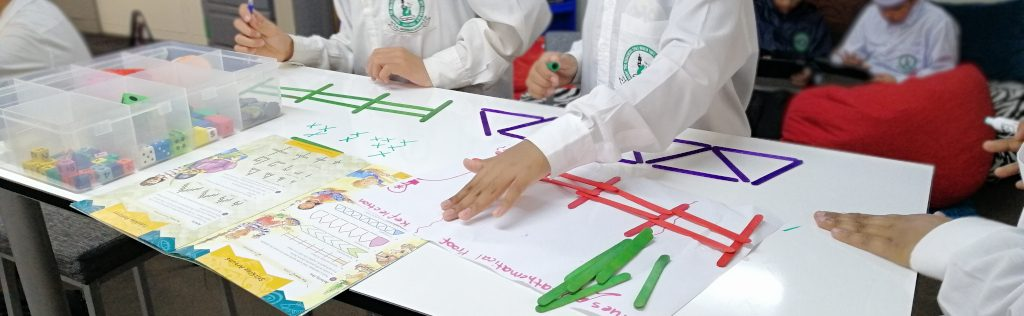# MathematicsMathematics is the exploration and use of patterns and relationships in quantities, space, and time. Statistics is the exploration and use of patterns and relationships in data.

By studying mathematics and statistics, students develop the ability to think creatively, critically, strategically, and logically. They learn to estimate with reasonableness, calculate with precision, and understand when results are precise and when they must be interpreted with uncertainty.

Mathematics and statistics have a broad range of practical applications in everyday life, in other learning areas, and in workplaces.

The Mathematics and Statistics course is divided into three strands.

– Number and algebra
– Geometry and measurement
– Statistics

https://nzcurriculum.tki.org.nz/The-New-Zealand-Curriculum/Mathematics-and-statistics